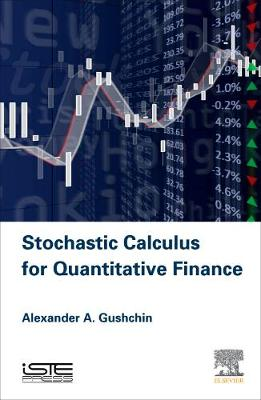•# Stochastic Calculus for Quantitative Finance (Hardback)

(author)
£76.00
Hardback 208 Pages / Published: 19/08/2015
• We can order this

Usually dispatched within 3 weeks

In 1994 and 1998 F. Delbaen and W. Schachermayer published two breakthrough papers where they proved continuous-time versions of the Fundamental Theorem of Asset Pricing. This is one of the most remarkable achievements in modern Mathematical Finance which led to intensive investigations in many applications of the arbitrage theory on a mathematically rigorous basis of stochastic calculus. Mathematical Basis for Finance: Stochastic Calculus for Finance provides detailed knowledge of all necessary attributes in stochastic calculus that are required for applications of the theory of stochastic integration in Mathematical Finance, in particular, the arbitrage theory. The exposition follows the traditions of the Strasbourg school. This book covers the general theory of stochastic processes, local martingales and processes of bounded variation, the theory of stochastic integration, definition and properties of the stochastic exponential; a part of the theory of Levy processes. Finally, the reader gets acquainted with some facts concerning stochastic differential equations.

Publisher: ISTE Press Ltd - Elsevier Inc
ISBN: 9781785480348
Number of pages: 208
Weight: 450 g
Dimensions: 229 x 152 x 13 mm

MEDIA REVIEWS
"...an excellent book which can be recommended for advanced courses in stochastic calculus, in particular, for courses in stochastic financial modelling (another name of quantitative finance)." --Zentralblatt MATH "The main goal of this short book is to introduce stochastic integration with respect to general semimartingales." --Mathematical Reviews